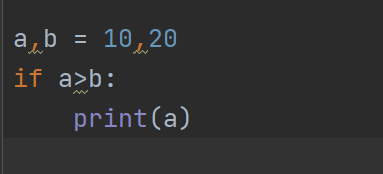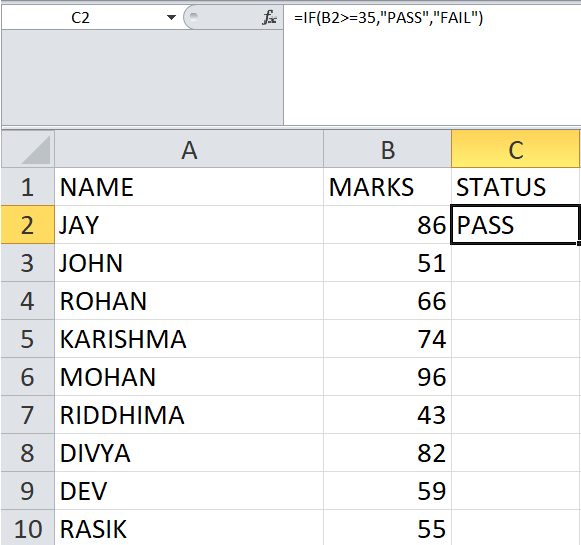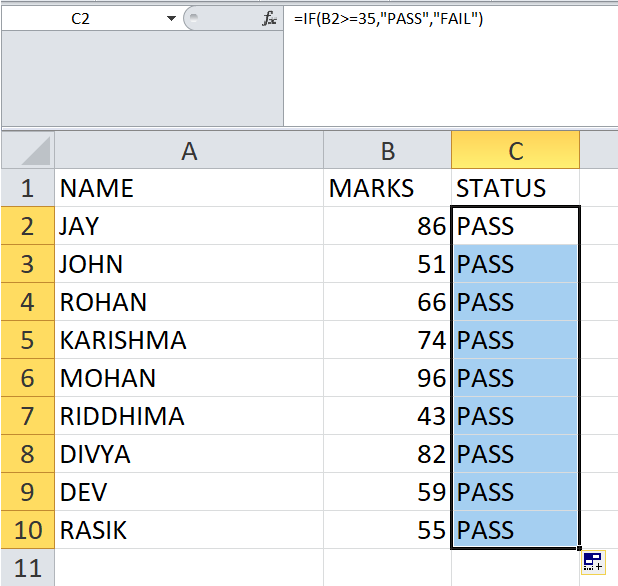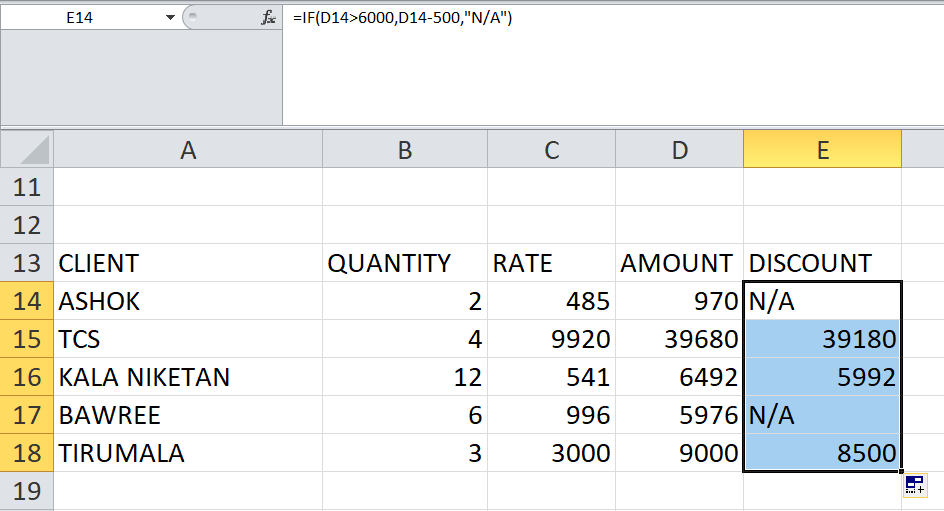# IF Function in Excel With Examples

• Last Updated : 13 Apr, 2021

The IF function is one of the most used, if not the most used function in Excel. It helps perform a single or a series of logical comparisons between entities. It works using the same principles as IF-ELSE conditional statements, used in most programming languages. Values satisfying the given condition, result in a positive output, while values not satisfying the given condition, result in a negative output.Python Code (For reference)

The IF function serves a single purpose, i.e., to run tests for specific conditional statements. It has the following syntax:

`Syntax: =IF(logical_argument, [value_if_true], [value_if_false])`

Return Value: IF function will return the values, “TRUE” or “FALSE” or the values specified by the user to be used in place of TRUE and FALSE.

Parameters:

The term argument refers to any expression that is enclosed inside the parenthesis of a function call.

The IF function in Excel consists of three main arguments. They are as follows:

1. logical_argument – This is the argument that defines the condition the user wants to check.

2. value_if_true – The value to be returned when the above condition returns TRUE (Optional, as default is TRUE).

3. value_if_false – The value to be returned when the above condition returns FALSE (Optional, as default is FALSE).

Example

Let’s say you want to find if all the students in a particular class PASSED or FAILED their tests. But, we can’t do that manually for 100 students. So, we can define the IF condition for one cell and apply it to all the cells we want.APPLYING TO ONE CELL

Now, let’s apply it to all the cells in the column.ALL CELLS

Now, let us try using IF with a bit more complex condition. Say, you have a list of clients, and they have made purchases from your store. Now, according to your store policy, you can provide a discount of Rs.500 to all clients that make purchases strictly above Rs.6,000. So, let’s add that condition to our sheet.Some clients are eligible for Discounts while some are not.

My Personal Notes arrow_drop_up